Question

# Give the appropriate form of the Henderson-Hasselbalch equation for an indicator and explain how the concentration...

Give the appropriate form of the Henderson-Hasselbalch equation for an indicator and explain how the concentration ratio determines the colour in solution and the transition interval.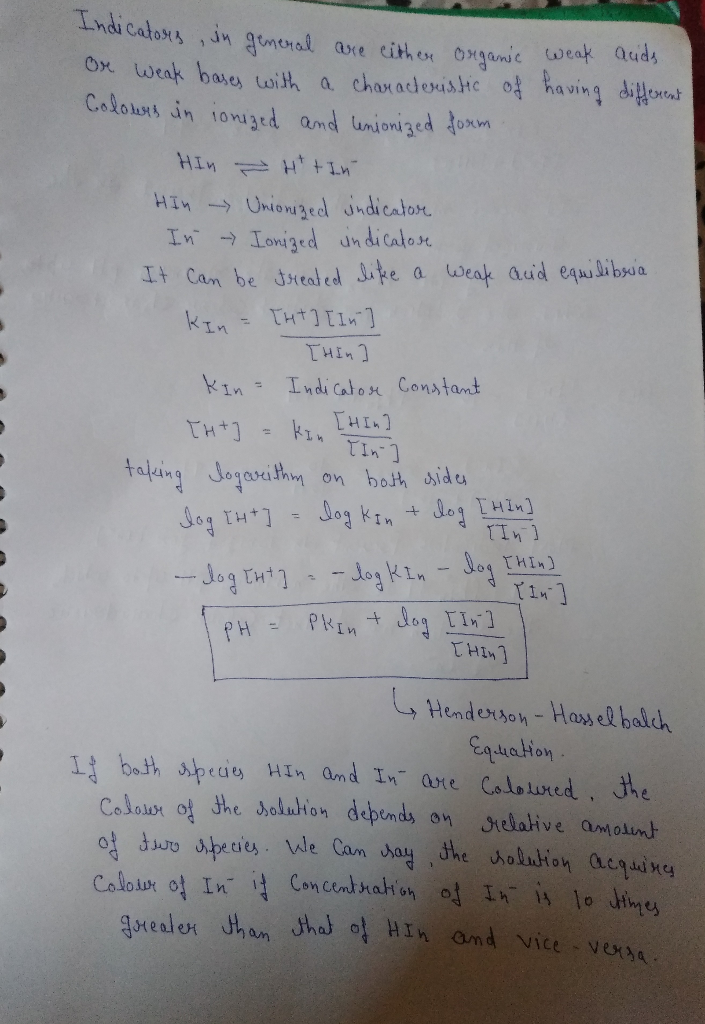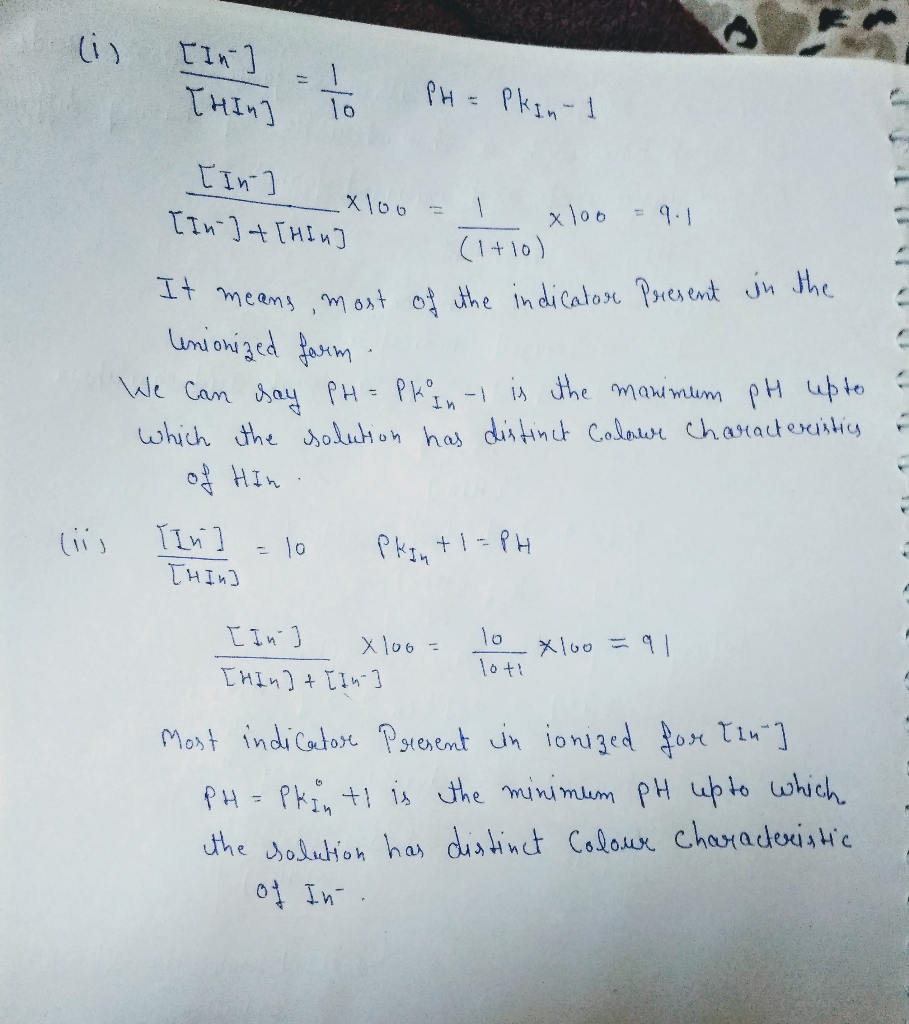#### Earn Coins

Coins can be redeemed for fabulous gifts.

Similar Homework Help Questions
• ### When is using the Henderson-Hasselbalch equation not appropriate for solving for pH? Why?

When is using the Henderson-Hasselbalch equation not appropriate for solving for pH? Why?

• ### According to the Henderson–Hasselbalch equation, when the pH of a solution of histidine is 1.85, what...

According to the Henderson–Hasselbalch equation, when the pH of a solution of histidine is 1.85, what is the ratio of the carboxylic acid form of the molecule to its conjugate base (the carboxylate form)? [For practice, either use Marvin Sketch or a piece of paper to draw the acid and conjugate base.] 1 or 4?

• ### The variable component of the Henderson-Hasselbalch equation relates the pH of a solution to: Select one:...

The variable component of the Henderson-Hasselbalch equation relates the pH of a solution to: Select one: a. The equilibrium constant of the weak acid. b. The inverse of the pOH. c. The log ratio of [dissociated form]/[acid]. d. The weak acid's pKa (This option ws wrong)

• ### The Henderson-Hasselbalch equation relates the pH of a buffer solution to the pKa of its conjugat...

The Henderson-Hasselbalch equation relates the pH of a buffer solution to the pKa of its conjugate acid and the ratio of the concentrations of the conjugate base and acid. The equation is important in laboratory work that makes use of buffered solutions, in industrial processes where pH needs to be controlled, and in medicine, where understanding the Henderson-Hasselbalch equation is critical for the control of blood pH. Part A As a technician in a large pharmaceutical research firm, you need...

• ### 10. Looking at the Henderson-Hasselbalch equation, what would be required to use the ratio as "[HA][A]"...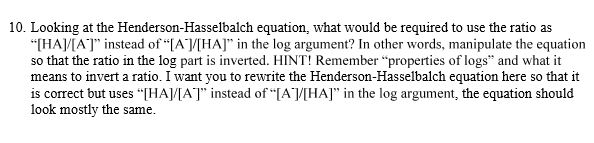10. Looking at the Henderson-Hasselbalch equation, what would be required to use the ratio as "[HA][A]" instead of "Ay[HA]" in the log argument? In other words, manipulate the equation so that the ratio in the log part is inverted. HINT! Remember "properties of logs" and what it means to invert a ratio. I want you to rewrite the Henderson-Hasselbalch equation here so that it is correct but uses "[HA][A]" instead of “[A][HA]" in the log argument, the equation should look...

• ### 1. Calculation: Using the Henderson-Hasselbalch equation, explain mathematically why a solution is at a pH below...

1. Calculation: Using the Henderson-Hasselbalch equation, explain mathematically why a solution is at a pH below the pKa for an acid that more than 50% of the molecules have the proton on (not off). 2. Calculation: If you have a pH of 5.5 for a weak acid with a pKa of 4.76, then is there more A- or more HA in the solution? Explain why in words using your knowledge of positive or negative log numbers.

• ### 5. (2pts) To calculate the pH of buffer solution we need to use Henderson-Hasselbalch equation The...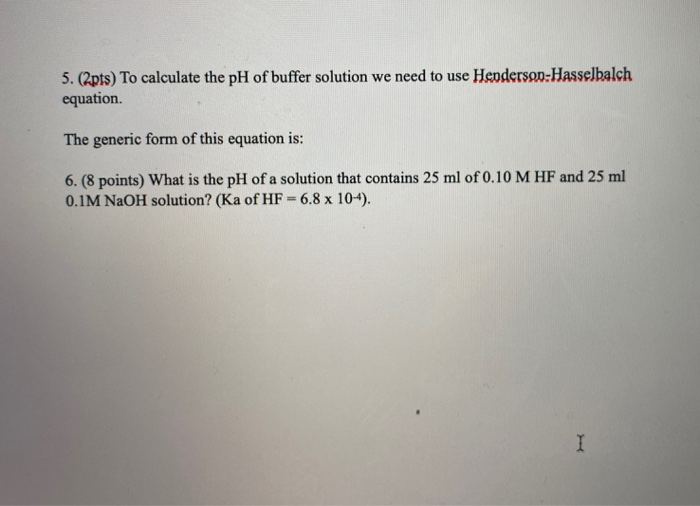5. (2pts) To calculate the pH of buffer solution we need to use Henderson-Hasselbalch equation The generic form of this equation is: 6. (8 points) What is the pH of a solution that contains 25 ml of 0.10 M HF and 25 ml 0.1M NaOH solution? (Ka of HF -6.8 x 104).

• ### Use the Henderson-Hasselbalch equation to calculate the pHpH of each solution: Part A a solution that...

Use the Henderson-Hasselbalch equation to calculate the pHpH of each solution: Part A a solution that is 0.19 MM in HCHO2HCHO2 and 0.10 MM in NaCHO2

• ### The Henderson-Hasselbalch equation connects pH to pk, by relating pH to the relative amounts of the...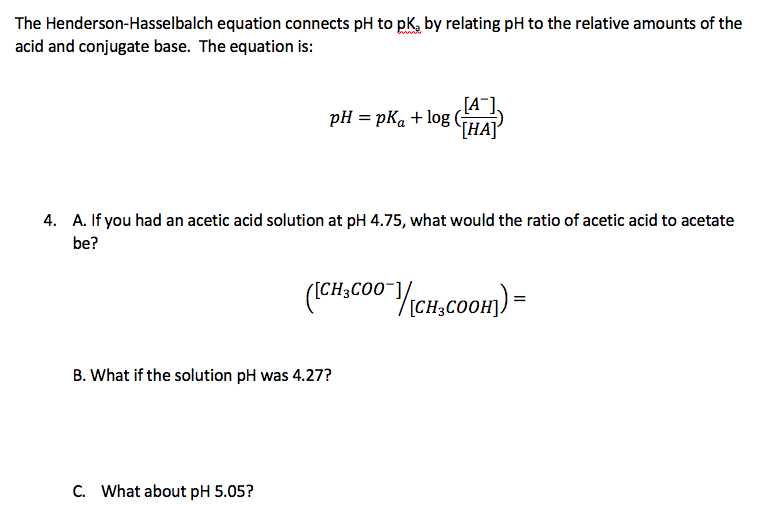The Henderson-Hasselbalch equation connects pH to pk, by relating pH to the relative amounts of the acid and conjugate base. The equation is: [A], pH = pKa + log [HA]' A. If you had an acetic acid solution at pH 4.75, what would the ratio of acetic acid to acetate 4. be? (Сн,соо у сн, соон) - ([CH3CO0¯], [CH3COOH], B. What if the solution pH was 4.27? C. What about pH 5.05?

• ### Use the Henderson-Hasselbalch equation to calculate the pH of each solution: A. a solution that is...

Use the Henderson-Hasselbalch equation to calculate the pH of each solution: A. a solution that is 0.14 M in HCHO2 and 0.13 M in NaCHO2 B. a solution that is 0.15 M in NH3 and 0.18 M in NH4Cl